Courses

# Triangles - Olympiad Level MCQ, Class 9 Mathematics

## 15 Questions MCQ Test Olympiad Preparation for Class 9 | Triangles - Olympiad Level MCQ, Class 9 Mathematics

Description
This mock test of Triangles - Olympiad Level MCQ, Class 9 Mathematics for Class 9 helps you for every Class 9 entrance exam. This contains 15 Multiple Choice Questions for Class 9 Triangles - Olympiad Level MCQ, Class 9 Mathematics (mcq) to study with solutions a complete question bank. The solved questions answers in this Triangles - Olympiad Level MCQ, Class 9 Mathematics quiz give you a good mix of easy questions and tough questions. Class 9 students definitely take this Triangles - Olympiad Level MCQ, Class 9 Mathematics exercise for a better result in the exam. You can find other Triangles - Olympiad Level MCQ, Class 9 Mathematics extra questions, long questions & short questions for Class 9 on EduRev as well by searching above.
QUESTION: 1

Solution:
QUESTION: 2

### In ΔABC if  ∠B = ∠ C = 45°, which of the following is the longest side ?

Solution: BC because the angles B and C are smaller than angle A which makes the side B and AC smaller than BC.
QUESTION: 3

### In a ΔABC if ∠ A = 45° and ∠B = 70° then the shortest and the largest sides of the triangle are :-

Solution:
QUESTION: 4

In Δ ABC if  ∠B = 45°, ∠ C = 65°, and the bisector of ∠BAC meets BC at P. Then the ascending order

of sides is :-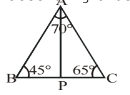Solution:
QUESTION: 5

In a ΔABC if 2∠ A = 3 ∠ B = 6 ∠ C then ∠ A ∠ B ∠ C are :

Solution:
QUESTION: 6

By which congruency property, the two triangles connected by the following figure are congruent :-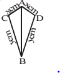Solution: By SSS rule...they r congruent CB=DB (given)AC=AD (given)AB =AB(common)
QUESTION: 7

In ΔABC, AB = AC and AD is perpendicular to BC. State the property by which ΔADB ≌ADC :-

Solution:
QUESTION: 8

State the property by which ΔADB ≌ADC in the following figure :-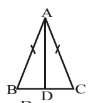Solution:
QUESTION: 9

In the given figure if AD = BC and AD || BC, then :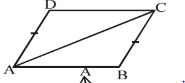Solution: The given figure is a parallelogram because AD=AB. Since,opposite sides of parallelogram are equal and also parallel. Therefore,AB will be equal to DC.
QUESTION: 10

In the figure given below, find the measure of the angles denoted by x,y, z,p,q and r.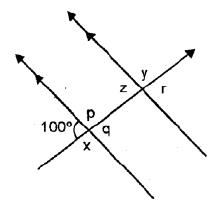Solution:

x = 180 - 100 [L.P. of angles] = 80°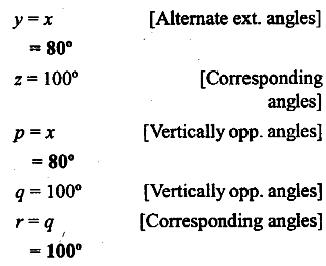QUESTION: 11

An exterior angle of a triangle is equal to the sum of two _________ angles :-

Solution: A related theorem. Because an exterior angle is equal to the sum of the opposite interior angles, it follows that it must be larger than either one of them. Stated more formally: Theorem: An exterior angle of a triangle is always larger then either opposite interior angle.
QUESTION: 12

In the following, the set of measures which can form a triangle :-

Solution:
QUESTION: 13

Sum of any two sides of a triangle is always __________ third side in a triangle :-

Solution:
QUESTION: 14

Can 90°, 90° and 20° form a triangle ?

Solution:
QUESTION: 15

In the given figure it is given that AB = CF, EF = BD and Ð AFE = Ð DBC. Then DAFE congruent to DCBD by which criterion ?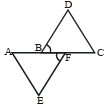Solution: